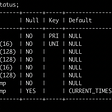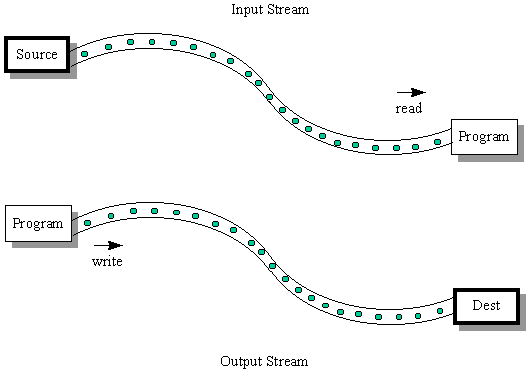# Map & Curry

`const sumCurried = a => b => a + bconst sumPartial = sumCurried(2)const arr = [4, 7, 8, 2, 10]const newarr = map(sumPartial, arr)// newarr: [6, 9, 10, 4, 12]`
`// map: function_signature, arraycase 0xaaaaaaaa {  let internal_fsig, fsig_size := getfSig(input_ptr)  let array_length := mload(add(input_ptr, fsig_size))  let values_ptr := add(add(input_ptr, fsig_size), 32)  // build returned array - add length  mstore(output_ptr, array_length)  // internal_output_ptr is the memory pointer  // at which the next array element is stored  let internal_output_ptr := add(output_ptr, 32)  result_length := 32  for { let i:= 0 } lt(i, array_length) { i := add(i, 1) } {    // Execute function on array element    let interm_res_length := executeInternal(      internal_fsig,      values_ptr,      32,      internal_output_ptr,      virtual_fns    )  // Move array values pointer & the resulting array pointer    // to the next element    values_ptr := add(values_ptr, 32)    internal_output_ptr := add(internal_output_ptr, interm_res_length)  // Update final result length    result_length := add(result_length, interm_res_length)  }}`
`0xffffffffcccccccc0000000200000028000000c4bbbbbbbbeeeeeeee0000000000000000000000000000000000000000000000000000000000000002aaaaaaaa00000000000000000000000000000000000000000000000000000000000000050000000000000000000000000000000000000000000000000000000000000004000000000000000000000000000000000000000000000000000000000000000700000000000000000000000000000000000000000000000000000000000000080000000000000000000000000000000000000000000000000000000000000002000000000000000000000000000000000000000000000000000000000000000a`
`ffffffff - execute signature (main entry point)cccccccc - recursive apply signature00000002 - number of steps for recursive apply00000028 - length in bytes for the first step000000c4 - length in bytes for the second stepbbbbbbbb - first step: curry function signatureeeeeeeee - sum function signature 0000000000000000000000000000000000000000000000000000000000000002   - partially applied argument for sum: 2 aaaaaaaa - second step: map signature 0000000000000000000000000000000000000000000000000000000000000005   - array length 0000000000000000000000000000000000000000000000000000000000000004 0000000000000000000000000000000000000000000000000000000000000007 0000000000000000000000000000000000000000000000000000000000000008 0000000000000000000000000000000000000000000000000000000000000002 000000000000000000000000000000000000000000000000000000000000000a   - array values`
`0x000000000000000000000000000000000000000000000000000000000000000500000000000000000000000000000000000000000000000000000000000000060000000000000000000000000000000000000000000000000000000000000009000000000000000000000000000000000000000000000000000000000000000a0000000000000000000000000000000000000000000000000000000000000004000000000000000000000000000000000000000000000000000000000000000c`
`0000000000000000000000000000000000000000000000000000000000000005    - array length 0000000000000000000000000000000000000000000000000000000000000006 0000000000000000000000000000000000000000000000000000000000000009 000000000000000000000000000000000000000000000000000000000000000a 0000000000000000000000000000000000000000000000000000000000000004 000000000000000000000000000000000000000000000000000000000000000c    - new array values`
`const sumCurried = a => b => a + bconst sumPartial = sumCurried(2)const arr = [4, 7, 8, 2, 10]const newarr = map(sumPartial, arr)// newarr: [6, 9, 10, 4, 12]`

# Reduce

`const sum = (a, b) => a + bconst arr = [4, 7, 8, 2, 10]const result = reduce(sum, arr, 0)// result: 31`
`// reduce: function_signature, array, accumulator (initial value)case 0x99999999 {  let internal_fsig, fsig_size := getfSig(input_ptr)  let new_ptr := add(input_ptr, fsig_size)  // The accumulator is treated as a uint256 for simplicity  let accumulator := mload(new_ptr)  new_ptr := add(new_ptr, 32)  let array_length := mload(new_ptr)  let values_ptr := add(new_ptr, 32)  for { let i:= 0 } lt(i, array_length) { i := add(i, 1) } {    // Store arguments in a temporary memory pointer, for simplicity    let temporary_input_ptr := 6000    mstore(temporary_input_ptr, accumulator)    mstore(add(temporary_input_ptr, 32), mload(values_ptr))    // Output memory pointer is 0x00    let interm_res_length := executeInternal(      internal_fsig,      temporary_input_ptr,      64,      0,      virtual_fns    )    // Read result from the output pointer    accumulator := mload(0)    // Move array values pointer to the next element    values_ptr := add(values_ptr, 32)  }  mstore(output_ptr, accumulator)  result_length := 32}`
`0xffffffffcccccccc00000001000000e899999999eeeeeeee000000000000000000000000000000000000000000000000000000000000000000000000000000000000000000000000000000000000000000000000000000050000000000000000000000000000000000000000000000000000000000000004000000000000000000000000000000000000000000000000000000000000000700000000000000000000000000000000000000000000000000000000000000080000000000000000000000000000000000000000000000000000000000000002000000000000000000000000000000000000000000000000000000000000000a`
`ffffffff - execute signature (main entry point)cccccccc - recursive apply signature00000001 - number of steps for recursive apply000000e8 - length in bytes for the first step99999999 - first step: reduce function signatureeeeeeeee - sum signature (reduce function argument) 0000000000000000000000000000000000000000000000000000000000000000    - reduce initial accumulator value 0000000000000000000000000000000000000000000000000000000000000005    - array length 0000000000000000000000000000000000000000000000000000000000000004 0000000000000000000000000000000000000000000000000000000000000007 0000000000000000000000000000000000000000000000000000000000000008 0000000000000000000000000000000000000000000000000000000000000002 000000000000000000000000000000000000000000000000000000000000000a    - array values`
`0x000000000000000000000000000000000000000000000000000000000000001f`

# Taylor Array Casting Example

`0xfffffffe00000005777777880300000026ee000003000000070000000e000000161100000300000411000003000002220000043333331c0000001f3333331a000000030203003333332800000002040533333332000000020601`
`fffffffe - store graph signature00000005 - length in bytes for the type definition head77777788 - this graph's signature03 - steps count00000026 - length in bytes for hardcoded graph inputsee000003 - tuple of 3 elements follows00000007 - additive sums of lengths for each tuple element0000000e0000001611000003 - type uint24 for slice_size - 02000004 - slice_size value11000003 - type uint24 for index - 03000002 - index value22000004 - type bytes4 for cast signature3333331c - cast function signature0000001f - length in bytes for graph steps3333331a - selectraw signature00000003 - how many inputs selectraw will receive02       - index for finding slice_size in all graph-local variables03       - index for finding index00       - index for to_type33333328 - curry signature0000000204       - index for cast signature05       - index for selectraw signature33333332 - map signature0000000206       - index for curry signature01       - index for the array to cast from`
`0xffffffff77777788ee0000020000000c000000202200000844000003110000204400000312000004000000020000000500000004`
`ffffffff - execute function signature, the main entry point77777788 - our array casting graph signatureee000002 - tuple of 2 elements following0000000c - additive sum of lengths in bytes for each tuple element0000002022000008 - type bytes8 for to_array44000003 - to_array signature: uint2561100002044000003 - actual from_array starts here, type int3212000004000000020000000500000004`
`0xee000001000000684400000311000020000000000000000000000000000000000000000000000000000000000000000200000000000000000000000000000000000000000000000000000000000000050000000000000000000000000000000000000000000000000000000000000004`
`ee000001 - all inputs & outputs are wrapped in a tupple0000006844000003 - final array uint25611000020000000000000000000000000000000000000000000000000000000000000000200000000000000000000000000000000000000000000000000000000000000050000000000000000000000000000000000000000000000000000000000000004   - array values: [2, 5, 7], left-padded to fit uint256`

# Full Code

--

--

--

## More from Loredana Cirstea

Building bricks for the World Computer #ethereum #Pipeline #dType #EIP1900 https://github.com/loredanacirstea, https://www.youtube.com/c/LoredanaCirstea

Love podcasts or audiobooks? Learn on the go with our new app.

## Streamlining Code Merge Process using CodeCommit Approval Rule Template## AWS ETL: Insert data to a relational database using Glue Job## Media Player Classic Home Cinema 1.9.18 [MPC HC]## Buna Ziua! OLX Group is now hiring remotely all across Romania## REST API Test Framework for Humans by Plain Text Files## Working with Streams and Optionals in Java 8## Building A Basic Web Scraper## Loredana Cirstea

Building bricks for the World Computer #ethereum #Pipeline #dType #EIP1900 https://github.com/loredanacirstea, https://www.youtube.com/c/LoredanaCirstea

## How to Deploy ArangoDB Graphs on GPUs for Accelerated Graph Algorithms using Nvidia’s RAPIDS…## Interacting with LND gRPC and REST APIs## How To Install TensorFlow Version 2.8 on The M1 chip Macbook With Ease## The AMM Book — production setup